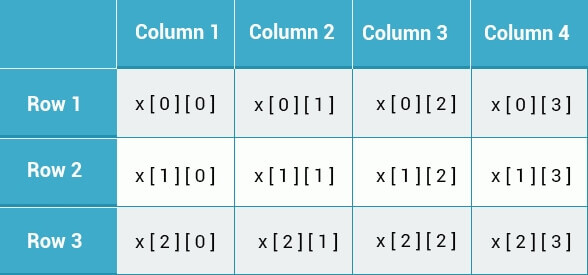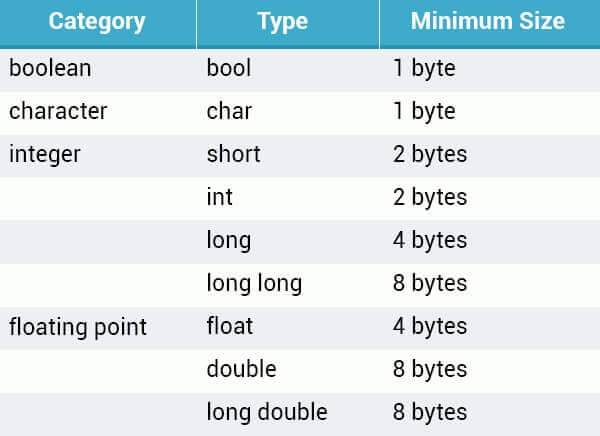# C++ 入门教程之三 -- 数据类型，数组及指针

### 介绍数据类型

``````55+15 //  legal C++ expression
``````

``````55 + "John" // illegal

``````

Boolean 类型只有两个可能的值：`true``false`

### 整型

``````int a = 42;
``````

``````signed int a;
``````

``````unsigned int a;
``````

short 型，一般默认空间占用大小，就是 2 个字节：

``````short int a;
``````

long 型，2倍默认空间大小：

``````long int a;
``````

### 浮点型

``````float a = 3.14;
``````

### 字符串

string 字符串类型是一个字符、数字和符号的集合，使用双引号来标记。

``````#include <string>
using namespace std;

int main() {
string test = "hello";
return 0;
}``````

### char 类型

char 类型包含了 1 个字节的整数，但是解释器会将这个整数解释为 ASCII 码对应字符。使用单引号来标记，如：

``````char a = 'a';
``````

### 变量定义规则

• 变量名首位必须是一个字母或下划线 `_`
• 首字符之后的可以使用字母或数字，不能使用特殊字符或空格

• 首字母及每个单词的首字符大写，如: BlankWord
• 首字母小写后续的每个单词首字符大写，如：blankWord

### 数组

``````int a = {1, 2, 5, 7, 8};
``````

``````int a[] = {1, 3, 7, 9};
``````

``````int b[] = {11, 45, 62, 70, 88};

cout << b << endl;
// Outputs 11

cout<< b << endl;
// Outputs 70``````

``````int myArr;

for(int x=0; x<5; x++) {
myArr[x] = 42;
}``````

### 多维数组

``````int a;
````````````int x = {
{2, 3, 4}, // 1st row
{8, 9, 10} // 2nd row
};``````

``````int x = {{2, 3, 4}, {8, 9, 10}};
``````

``````int x = {{2, 3, 4}, {8, 9, 10}};
cout << x << endl;

//Outputs 4``````

### 指针

``````int score = 5;
cout << &score << endl;

//Outputs "0x29fee8"``````

``````int *ip;  // pointer to an integer
double *dp;   // pointer to a double
float *fp;  // pointer to a float
char *ch;  // pointer to a character``````

``````int score = 5;
int *scorePtr;
scorePtr = &score;

cout << scorePtr << endl;

//Outputs "0x29fee8"``````

scorePtr 的值就是 score 变量的内存地址。

• 地址查询符`&`，返回操作数所在内存地址 referencing
• 数据查询符`*`，返回操作数的内存地址下的数据 dereferencing

``````int var = 50;
int  *p;
p = &var;

cout << var << endl;
// Outputs 50 (the value of var)

cout << p << endl;
// Outputs 0x29fee8 (var's memory location)

cout << *p << endl;
/* Outputs 50 (the value of the variable
stored in the pointer p) */``````

``````int x = 5;
int *p = &x;

x = x + 4;
x = *p + 4;
*p = *p + 4;``````

P 是 x 的指针，以上示例的三种运算结果都是一样的，都是将变量 x 的值进行计算。我们可以通过定义变量的指针来操作变量值。

### 静态内存和动态内存

• the stack 静态内存空间：所有的本地变量占用静态内存空间
• the heap 动态内存空间：再程序运行时动态进行分配的空间，默认不占用

``````new int;
``````

``````int *p = new int;
*p = 5;``````

``````int *p = new int; // request memory
*p = 5; // store value

cout << *p << endl; // use value

delete p; // free up the memory``````

``````int *p = new int; // request memory
*p = 5; // store value

delete p; // free up the memory
// now p is a dangling pointer

p = new int; // reuse for a new address``````

``````int *ptr = NULL;
``````

``````int *p = NULL; // Pointer initialized with null
p = new int; // Request memory
delete [] p; // Delete array pointed to by p``````

### 数据大小``````sizeof(int)
``````

``````cout << "char: " << sizeof(char) << endl;
cout << "int: " << sizeof(int) << endl;
cout << "float: " << sizeof(float) << endl;
cout << "double: " << sizeof(double) << endl;
int var = 50;
cout << "var: " << sizeof(var) << endl;

/* Outputs
char: 1
int: 4
float: 4
double: 8
var: 4
*/ ``````

``````double myArr;
cout << sizeof(myArr) << endl;

//Outputs 80``````

``````int numbers;
cout << sizeof(numbers) / sizeof(numbers);

// Outputs 100``````